# Foundations, Sorting on a Linear Array, Algorithm Assessment

Tom Kelliher, CS 315

Jan. 25, 1999

### From Last Time

Foundations: asymptotic analysis, mathematical induction.

### Outline

1. Foundations: loop invariants.

2. Sorting on a linear array: linear arrays, sorting, time analysis.

3. Algorithm assessment.

### Coming Up

Using fewer processors, the bit model, lower bounds.

# Foundations

## Loop Invariants

Four characteristics:

1. Must be true upon entry into the loop
2. Execution of the loop body must preserve the invariant
3. Invariant must capture the correctness and the meaning of the loop
4. Loop must terminate

Consider the following sorting algorithm which sorts the n elements of data (the elements are indexed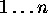):

```      for i := n downto 2 do
largest := i;

for j := i-1 downto 1 do
if data[j] > data[largest]
largest := j;

swap(data[largest], data[i]);
```

Prove that the algorithm sorts the elements of the array data into ascending order.

First, demonstrate the algorithm on the array

```int data[] = { 12, 19, 6, 15 };
```

# Sorting on a Linear Array

## Linear Arrays

A linear array: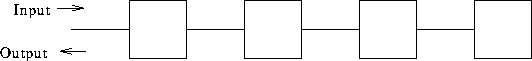Cell properties:

1. Local program: a ``few'' operations.

2. Local storage: a ``few'' words of data.

3. Operation sequence:

3. Perform computation indicated by local program.

4. Generate output for neighbors.

5. Update local storage.

4. Synchronized to a global clock.

5. Must all the programs be identical? Elegance?

Communication properties:

2. Left, right neighbors.

3. Fixed connection network.

4. Data pulsing through network: systolic computation.

## Sorting

1. Phase 1: sorting program.
1. Accept input from left neighbor.

2. Compare to stored value.

3. Output larger value to right neighbor.

4. Store smaller value.

2. Phase 2: output. Begin passing left when no more input is received from the left neighbor.

Example: sort 12, 19, 6, 15.

How many steps to sort? How many steps to output?

# Algorithm Assessment

How can we measure a parallel algorithm? Apply following to sorting on the linear array.

1. Run time: T.

2. Number of processors: N.

3. Speedup: Let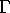be the running time of the fastest sequential algorithm. Then:What is linear speedup?

Say you have P processors. Speedup is at most P. Why? (Hint: a parallel algorithm that runs in T steps on P processors can be simulated on a sequential machine in how many steps?)

4. Work: W = TP.

Measures algorithm inefficiencies due to idle processors.

5. Efficiency of processor utilization: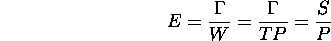6. Is it always possible to minimize T and E?

Example: consider two algorithms for solving a problem of size M:

1. M steps on an M processor machine.

2.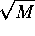steps on an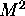processor machine.

If we have anprocessor machine and need to run the algorithm X times, which algorithm should we use? Assume theprocessor machine may be split in M M processor machines.

(Recall: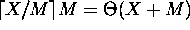.)

Thomas P. Kelliher
Mon Jan 25 09:49:26 EST 1999
Tom Kelliher